# A.Richter

•### Geometry

Book

A.Richter

•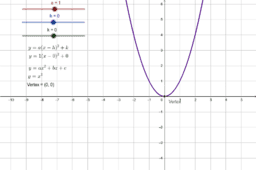Book

A.Richter

•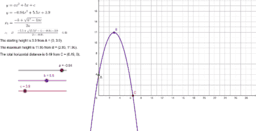### Trajectory of an Object in the Air

Activity

A.Richter

•### Finding the Maximum or Minimum

Activity

A.Richter

•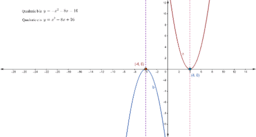### Maximum or Minimum of a Quadratic

Activity

A.Richter

•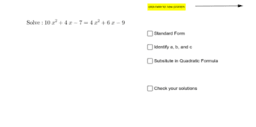### Practice - Using the Quadratic Formula to Find Roots

Activity

A.Richter

•### Practice - Factoring to Find Roots

Activity

A.Richter

•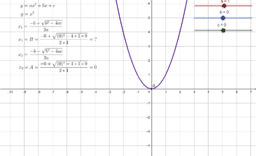### Finding the Roots of a Quadratic Equation

Activity

A.Richter

•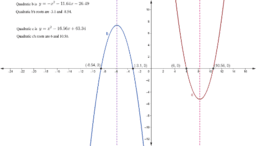### The Roots of Two Quadratic Equations

Activity

A.Richter

•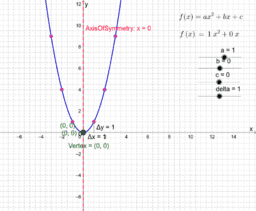### A Quadratic and Its Data Table

Activity

A.Richter

•### The Graph of a Quadratic Equation

Activity

A.Richter

•### Lines

Book

A.Richter

•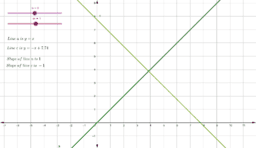### Equations for Two Perpendicular Lines

Activity

A.Richter

•### Equations for Two Parallel Lines

Activity

A.Richter

•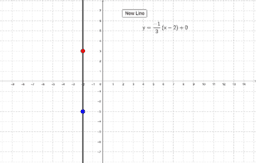### Graph the Line - Level 3

Activity

A.Richter

•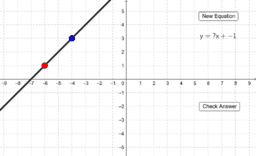### Graph the Line - Level 2

Activity

A.Richter

•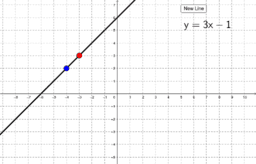### Graph the Line - Level 1

Activity

A.Richter

•### The Point-Slope Form of a Linear Equation

Activity

A.Richter

•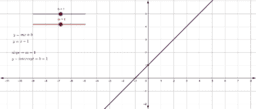### The Slope-Intercept Form of a Linear Equation

Activity

A.Richter

•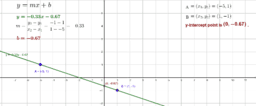### The Slope of a Line and the y-Intercept Equation

Activity

A.Richter

•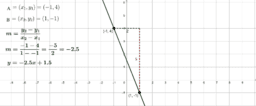### Exploring the Slope of a Line

Activity

A.Richter

•### Distance between Two Points - Basic

Activity

A.Richter

•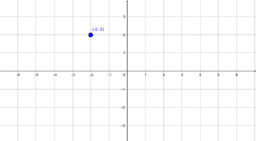### X-Y Coordinate Plane

Book

A.Richter

•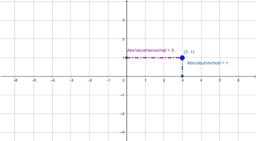Activity

A.Richter